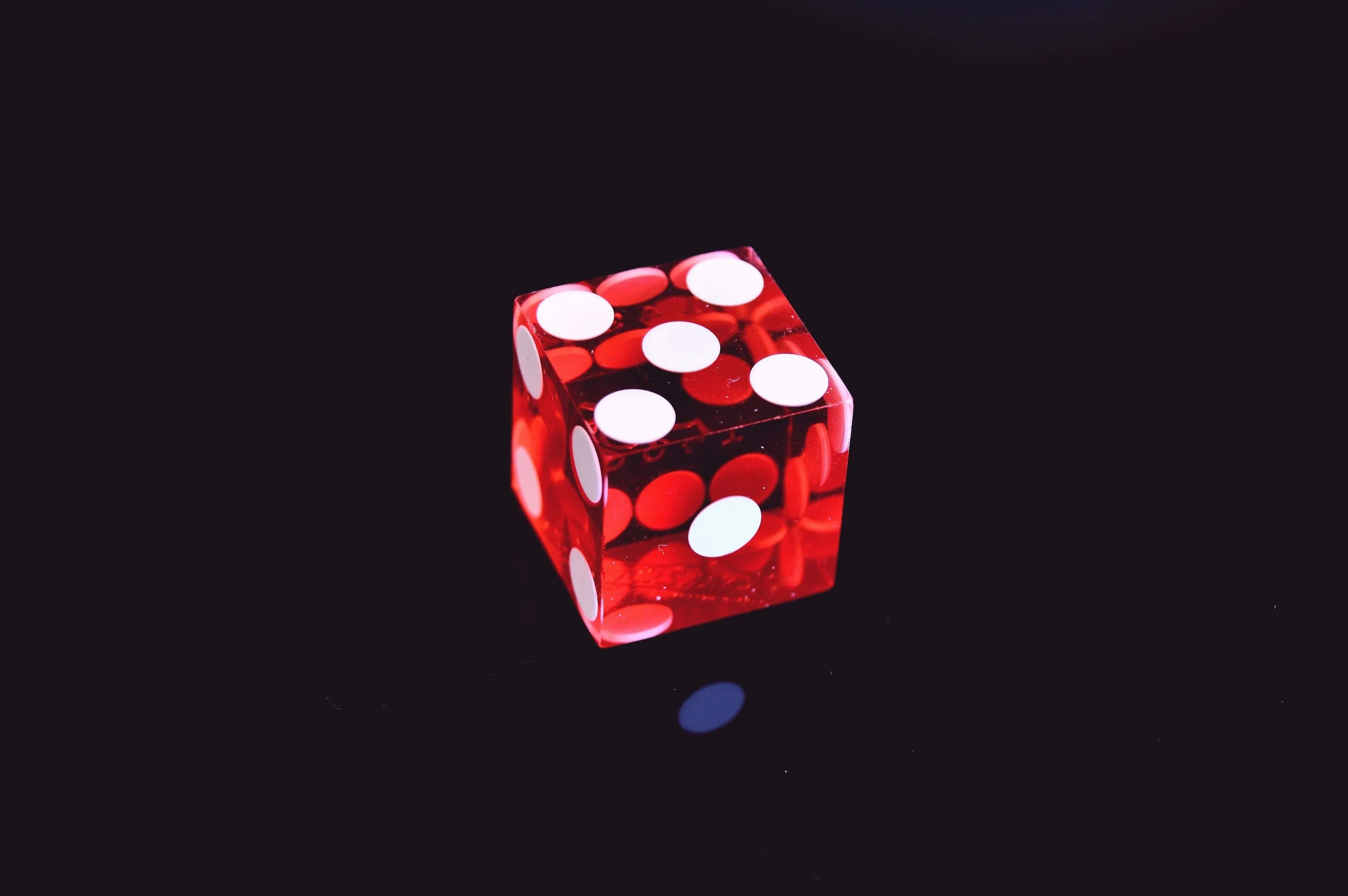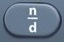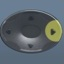# ProbabilityBasics
Probability is the chance that an event will occur.

Probability can be expressed as a fraction, decimal, or percent.

Probability of Simple Event =$\bf\displaystyle\frac{Number\,of\,Events}{Number\,of\,Possible\,Events}$
• The TI-30XS MultiView Scientific Calculator may or may not be needed here.
• Calculator Not Needed: Probability of rolling a five on a single die =$\bf\displaystyle\frac{1}{6}$
• Calculator Needed: Probability of picking 12 orange balls out of a total of 100 balls, the rest of which are not orange =$\bf\displaystyle\frac{12}{100}$ =$\bf\displaystyle\frac{3}{25}$
• When asked to convert a fraction to decimal form, use the toggle button.

Probability of Compound Event = Probability of Multiple Events
• The calculator is usually needed here.
• To arrive at the answer, multiply the probabilities.
• When fractions are multiplied, the answer will be in fraction form.
-> Probability of rolling 1 followed by 2 with a die  =$\bf\displaystyle\frac{1}{6}$ ×$\bf\displaystyle\frac{1}{6}$ =$\bf\displaystyle\frac{1}{36}$
• When percents are multiplied, the answer will be in decimal form.
-> Probability of snow 40% on Monday followed by 60% on Tuesday = 40% × 60% = 0.24
• When asked to convert a decimal to percent frome, move the decimal point two places to the right.
-> 0.24 = 24.% = 24%

Formulas (NOT on Mathematics Formula Sheet)

Probability of Simple Event =$\bf\displaystyle\frac{Number\,of\,Events}{Number\,of\,Possible\,Events}$

Probability of Compound Event = Probability of Multiple Events

Question
When rolling a single die from a pair of dice, there are six possible outcomes: 1 or 2 or 3 or 4 or 5 or 6.  In fractional terms, when a die is rolled, what is the probability of a 2?$\bf\displaystyle\frac{1}{6}$

What is the probability of a simple event?
What is the probability of one out of six possible events?
Calculator not needed.$\bf\displaystyle\frac{1}{6}$

Question
In fractional terms, what is the probability of picking 24 orange balls out of a total of 100 balls, the rest of which are not orange?$\bf\displaystyle\frac{6}{25}$

What is the probability of a simple event?
What is the probability of 24 out of 100 possible events?
Calculator needed.$\bf\displaystyle\frac{6}{25}$

Input Display Commentblinker clears screen24100$\bf\displaystyle\frac{24}{100}$ Simple Event$\bf\displaystyle\frac{6}{25}$ Answer
###### Probability

Question
With reference to the question above, express your answer in decimal form.

0.24

See Toggle.$\bf\displaystyle\frac{6}{25}$ ⇔ 0.24

Input Display Commentblinker clears screen24100$\bf\displaystyle\frac{24}{100}$ Simple Event$\bf\displaystyle\frac{6}{25}$0.24 Answer
(Toggle)
###### Probability

Question

With reference to the question above, express your answer in percent form.

24%

See Percent.
To convert a decimal to percent, move the decimal point two places to the right.
0.24 = 24.% = 24%

Question
When rolling a single die from a pair of dice, there are six possible outcomes: 1 or 2 or 3 or 4 or 5 or 6.  In fractional terms, when a die is rolled, what is the probability of rolling a 2 followed by 5?$\bf\displaystyle\frac{1}{36}$

What is the probability of a compound event?
Multiply the probabilities.$\bf\displaystyle\frac{1}{6}$ ×$\bf\displaystyle\frac{1}{6}$ =$\bf\displaystyle\frac{1}{36}$

Input Display Commentblinker clears screen16×16$\bf\displaystyle\frac{1}{6}$*$\bf\displaystyle\frac{1}{6}$ Compound Event$\bf\displaystyle\frac{1}{36}$ Answer
###### Probability

Question
With reference to the question above, express your answer in decimal form.

0.027777778

See Toggle.$\bf\displaystyle\frac{1}{36}$ ⇔ 0.027777778

Input Display Commentblinker clears screen16×16$\bf\displaystyle\frac{1}{6}$*$\bf\displaystyle\frac{1}{6}$ Compound Event$\bf\displaystyle\frac{1}{36}$0.027777778 Answer
(Toggle)
###### Probability

Question
With reference to the question above, express your answer in percent form.

2.777777778%

See Percent.
To convert a decimal to percent, move the decimal point two places to the right.
0.027777778 = 2.7777778%

Question

With reference to the question above, round your answer to the nearest hundredth.

2.78%

See Rounding.
2.7777778%  2.78%

Question

The probability of snow is 65% on Friday, 75% on Saturday, 55% on Sunday, and 85% on Monday.  In percent terms, rounded to the nearest tenth, what is the probability of snow on all four days?

22.8%

What is the probability of a compound event?
Multiply the probabilities.
65% × 75% × 55% × 85% = 0.22790625
To convert a decimal to percent, move the decimal point two places to the right.
0.22790625 = 22.790625% (See Percent.)
Round to the nearest tenth.

22.790625% 22.8% (See Rounding.)

Input Display Commentblinker clears screen
65×
75×
55×
85×
65%*75%*55%*85% Compound Event0.22790625 Answer
(before percent and rounding)
###### Probability

Practice – Questions
1.  When flipping a coin, there are two possible outcomes: heads or tails.  In fractional terms, when a coin is flipped, what is the probability of flipping heads?

2.  When flipping a coin, there are two possible outcomes: heads or tails.  In fractional terms, when a coin is flipped, what is the probability of flipping tails?

3.  When flipping a coin, there are two possible outcomes: heads or tails.  In fractional terms, when a coin is flipped, what is the probability of flipping heads followed by heads?

4.  When flipping a coin, there are two possible outcomes: heads or tails.  In fractional terms, when a coin is flipped, what is the probability of flipping tails followed by tails?

5.  When flipping a coin, there are two possible outcomes: heads or tails.  In fractional terms, when a coin is flipped, what is the probability of flipping heads followed by tails?

6.  When flipping a coin, there are two possible outcomes: heads or tails.  In fractional terms, when a coin is flipped, what is the probability of flipping tails followed by heads?

7.  When flipping a coin, there are two possible outcomes: heads or tails.  In decimal terms, when a coin is flipped, what is the probability of flipping heads followed by tails?

8.  When flipping a coin, there are two possible outcomes: heads or tails.  In percent terms, when a coin is flipped, what is the probability of flipping heads followed by tails?

9.  When flipping a coin, there are two possible outcomes: heads or tails.  In percent terms, rounded to the nearest tenth, when a coin is flipped, what is the probability of flipping heads followed by heads followed by heads followed by heads?

10.  The probability of precipitation is 55% on Friday, 65% on Saturday, and 45% on Sunday.  In percent terms, rounded to the nearest hundredth, what is the probability of precipitation on all three days?

1.$\bf\displaystyle\frac{1}{2}$

2.$\bf\displaystyle\frac{1}{2}$

3.$\bf\displaystyle\frac{1}{4}$

4.$\bf\displaystyle\frac{1}{4}$

5.$\bf\displaystyle\frac{1}{4}$

6.$\bf\displaystyle\frac{1}{4}$

7.  0.25

8.  25%

9.  6.3%

10.  16.09%